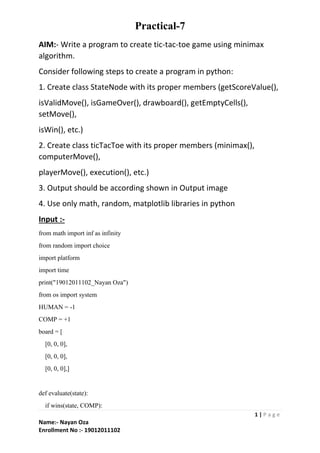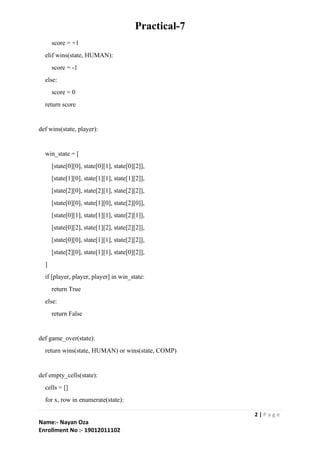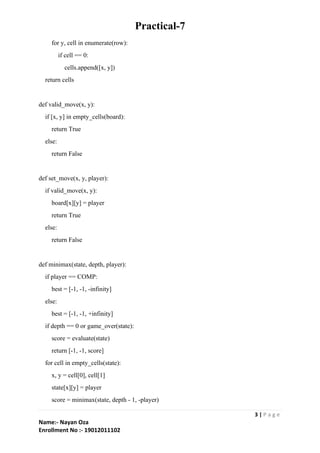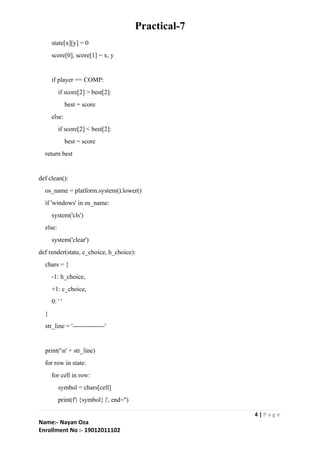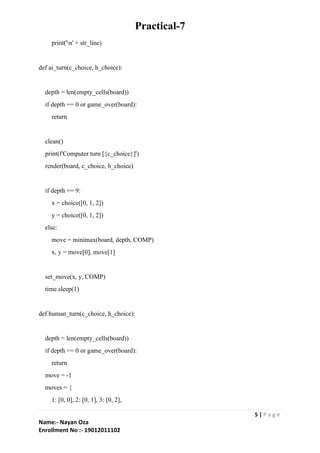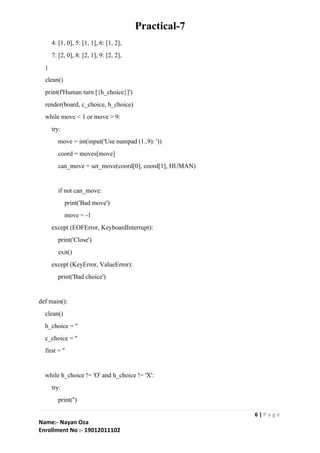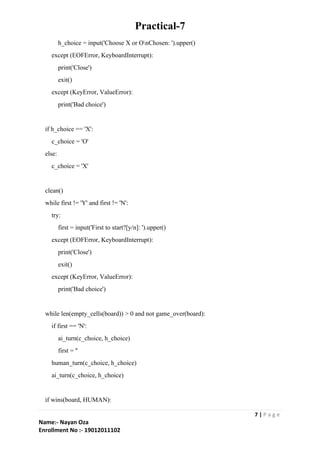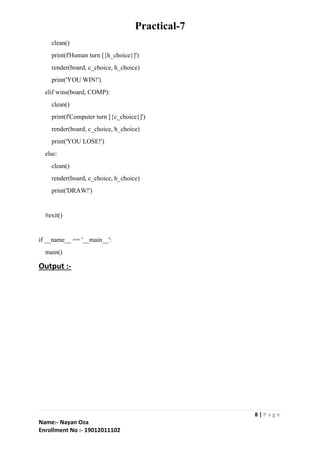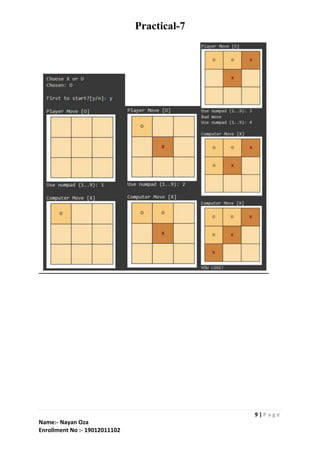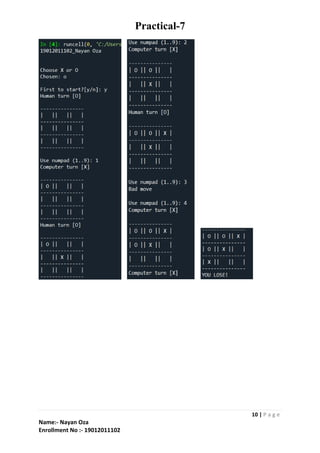Próximos SlideShares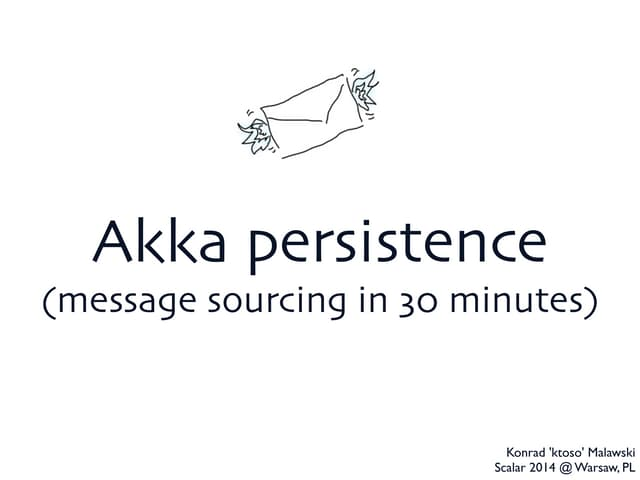Akka persistence == event sourcing in 30 minutes
Carregando em ... 3
1 de 10Akka persistence == event sourcing in 30 minutesKonrad MalawskiCARTOって何？CARTOでできることYu ImaiV1.1 CD03 Azure Active Directory B2C/B2B コラボレーションによる Customer Identity and Ac...junichi annoStep by Step Asp.Net GridView TutorialsNilesh kumar JadavKey recovery attacks against commercial white-box cryptography implementation...CODE BLUEゼロ・トラストネットワークを実現する、 マイクロソフトの新しいSecurityサービスの全貌 〜 SIEM、SOCの構築をサポートするMicrosoft ...ID-Based Security イニシアティブ

### 19012011102_Nayan Oza_Practical-7_AI.pdf

1. Practical-7 1 | P a g e Name:- Nayan Oza Enrollment No :- 19012011102 AIM:- Write a program to create tic-tac-toe game using minimax algorithm. Consider following steps to create a program in python: 1. Create class StateNode with its proper members (getScoreValue(), isValidMove(), isGameOver(), drawboard(), getEmptyCells(), setMove(), isWin(), etc.) 2. Create class ticTacToe with its proper members (minimax(), computerMove(), playerMove(), execution(), etc.) 3. Output should be according shown in Output image 4. Use only math, random, matplotlib libraries in python Input :- from math import inf as infinity from random import choice import platform import time print("19012011102_Nayan Oza") from os import system HUMAN = -1 COMP = +1 board = [ [0, 0, 0], [0, 0, 0], [0, 0, 0],] def evaluate(state): if wins(state, COMP):
2. Practical-7 2 | P a g e Name:- Nayan Oza Enrollment No :- 19012011102 score = +1 elif wins(state, HUMAN): score = -1 else: score = 0 return score def wins(state, player): win_state = [ [state, state, state], [state, state, state], [state, state, state], [state, state, state], [state, state, state], [state, state, state], [state, state, state], [state, state, state], ] if [player, player, player] in win_state: return True else: return False def game_over(state): return wins(state, HUMAN) or wins(state, COMP) def empty_cells(state): cells = [] for x, row in enumerate(state):
3. Practical-7 3 | P a g e Name:- Nayan Oza Enrollment No :- 19012011102 for y, cell in enumerate(row): if cell == 0: cells.append([x, y]) return cells def valid_move(x, y): if [x, y] in empty_cells(board): return True else: return False def set_move(x, y, player): if valid_move(x, y): board[x][y] = player return True else: return False def minimax(state, depth, player): if player == COMP: best = [-1, -1, -infinity] else: best = [-1, -1, +infinity] if depth == 0 or game_over(state): score = evaluate(state) return [-1, -1, score] for cell in empty_cells(state): x, y = cell, cell state[x][y] = player score = minimax(state, depth - 1, -player)
4. Practical-7 4 | P a g e Name:- Nayan Oza Enrollment No :- 19012011102 state[x][y] = 0 score, score = x, y if player == COMP: if score > best: best = score else: if score < best: best = score return best def clean(): os_name = platform.system().lower() if 'windows' in os_name: system('cls') else: system('clear') def render(state, c_choice, h_choice): chars = { -1: h_choice, +1: c_choice, 0: ' ' } str_line = '---------------' print('n' + str_line) for row in state: for cell in row: symbol = chars[cell] print(f'| {symbol} |', end='')
5. Practical-7 5 | P a g e Name:- Nayan Oza Enrollment No :- 19012011102 print('n' + str_line) def ai_turn(c_choice, h_choice): depth = len(empty_cells(board)) if depth == 0 or game_over(board): return clean() print(f'Computer turn [{c_choice}]') render(board, c_choice, h_choice) if depth == 9: x = choice([0, 1, 2]) y = choice([0, 1, 2]) else: move = minimax(board, depth, COMP) x, y = move, move set_move(x, y, COMP) time.sleep(1) def human_turn(c_choice, h_choice): depth = len(empty_cells(board)) if depth == 0 or game_over(board): return move = -1 moves = { 1: [0, 0], 2: [0, 1], 3: [0, 2],
6. Practical-7 6 | P a g e Name:- Nayan Oza Enrollment No :- 19012011102 4: [1, 0], 5: [1, 1], 6: [1, 2], 7: [2, 0], 8: [2, 1], 9: [2, 2], } clean() print(f'Human turn [{h_choice}]') render(board, c_choice, h_choice) while move < 1 or move > 9: try: move = int(input('Use numpad (1..9): ')) coord = moves[move] can_move = set_move(coord, coord, HUMAN) if not can_move: print('Bad move') move = -1 except (EOFError, KeyboardInterrupt): print('Close') exit() except (KeyError, ValueError): print('Bad choice') def main(): clean() h_choice = '' c_choice = '' first = '' while h_choice != 'O' and h_choice != 'X': try: print('')
7. Practical-7 7 | P a g e Name:- Nayan Oza Enrollment No :- 19012011102 h_choice = input('Choose X or OnChosen: ').upper() except (EOFError, KeyboardInterrupt): print('Close') exit() except (KeyError, ValueError): print('Bad choice') if h_choice == 'X': c_choice = 'O' else: c_choice = 'X' clean() while first != 'Y' and first != 'N': try: first = input('First to start?[y/n]: ').upper() except (EOFError, KeyboardInterrupt): print('Close') exit() except (KeyError, ValueError): print('Bad choice') while len(empty_cells(board)) > 0 and not game_over(board): if first == 'N': ai_turn(c_choice, h_choice) first = '' human_turn(c_choice, h_choice) ai_turn(c_choice, h_choice) if wins(board, HUMAN):
8. Practical-7 8 | P a g e Name:- Nayan Oza Enrollment No :- 19012011102 clean() print(f'Human turn [{h_choice}]') render(board, c_choice, h_choice) print('YOU WIN!') elif wins(board, COMP): clean() print(f'Computer turn [{c_choice}]') render(board, c_choice, h_choice) print('YOU LOSE!') else: clean() render(board, c_choice, h_choice) print('DRAW!') #exit() if __name__ == '__main__': main() Output :-
9. Practical-7 9 | P a g e Name:- Nayan Oza Enrollment No :- 19012011102
10. Practical-7 10 | P a g e Name:- Nayan Oza Enrollment No :- 19012011102